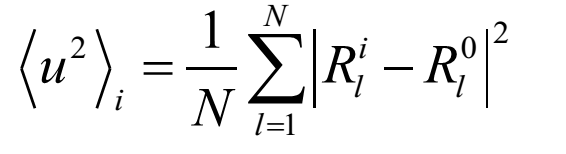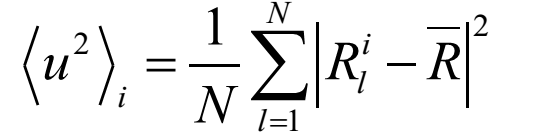# Definition of MSD in LAMMPS

Dear LAMMPS users,
I looked into the source file compute_msd.cpp, and it seems that MSD was defined as mean square displacement from the initial**(t=0)** position(I’m not quite sure because I have not understood the code entirely),Where l represents the atom l, i represents the ith configuration, Rl0 represents the initial position for atom l.
But in other situations, MSD was defined as mean square displacement from the average position,where l represents the atom l, i represents the ith configuration, the position is the average position for atom l.
For example, in Lattice vibrations in plutonium: Molecular dynamics calculation, MSD is defined in this way.
Did I understand the definition of MSD in LAMMPS rightly? If so, does this two different definition affects the value of MSD much? How can I compare my MSD results derived from LAMMPS with others defined in the second way?
Sincerely,
Lixin

This particular subject has been touched on many times in the mailing list archives. LAMMPS does not store positions from every time-step, so you do not get an average over all possible time windows. That being said, the starting point for extracting MSD is whenever you invoke the compute (your t=0). If you want to calculate true autocorrelation statistics for a stationary process in an efficient manner, it’s best done through post-processing.It is not very hard to show that in the limit of fully converged averages, the two definitions differ by a constant positive term = <(R-R0)^2>, which you could probably estimate, or have LAMMPS calculate it for you exactly.Sorry, I meant to write <(-R0)^2>.Righto. And another case of me responding with my gut instead of reading fully.